# Import TensorFlow Channel Feedback Compression Network and Deploy to GPU

This example shows how to import a pretrained TensorFlow™ channel state feedback autoencoder for generating GPU-specific C++ code. In this example, you learn how to import, test, and fine-tune the network weights to a new channel. Then you generate C++ code to target an NVIDIA® GPU using the cuDNN deep learning optimized library. Finally, you compare the prediction accuracy of the generated code from the network against the prediction accuracy in MATLAB®.

Generating optimized code for prediction using a high-performance library such as cuDNN improves latency, throughput, and memory efficiency by combining network layers and optimizing kernel selection. You can integrate the generated code into your project as source code, static or dynamic libraries, or executables that you can deploy to a variety of NVIDIA GPU platforms.

#### Third-Party Prerequisites

Required

This example generates CUDA MEX and has the following third-party requirements.

• CUDA® enabled NVIDIA GPU and compatible driver.

For details, see GPU Computing Requirements (Parallel Computing Toolbox).

Optional

For non-MEX builds that generates static, or dynamic libraries or executables, this example has the following additional requirements.

• NVIDIA toolkit

• NVIDIA cuDNN library

• Environment variables for the compilers and libraries

For more information, see Installing Prerequisite Products (GPU Coder) and Setting Up the Prerequisite Products (GPU Coder) for GPU Coder™.

### Import Pretrained Model

This example works with the CSINet TensorFlow models available at the GitHub repository in . The repository contains eight models trained on the COST2100 channel model for indoor and outdoor environments and compression rates $1/4$, $1/16$, $1/32$, and $1/64$. This example uses an outdoor environment model with a compression rate of $1/32$. To use another pretrained model from  with a different compression rate, download the model to the example folder and set the `modelDir` input argument to the downloaded model location.

Using a model with a lower compression rate, for example $1/4$, achieves higher prediction accuracy at the expense of larger memory footprint, longer training, and prediction time.

Using the `helperDownloadFiles` function, download the pretrained model from `https://ssd.mathworks.com/supportfiles/spc/importCSINetTensorFlow/tensorflowModel/CSINetOutdoor64.zip`. If you do not have an internet connection, you can download the ZIP file manually on a computer that is connected to the internet, unzip it, and save it to the example folder.

`helperDownloadFiles('pretrainedModel');`
```Starting download pretrained model. Downloading pretrained model. Extracting files. Extract complete. ```

Use `importNetworkFromTensorFlow` to import the pretrained CSINet model into the MATLAB workspace as a `dlnetwork` (Deep Learning Toolbox) object`.`

```modelDir = fullfile(pwd,"CSINetOutdoor64","CSINetOutdoor64"); importedCSINet = importNetworkFromTensorFlow(modelDir);```
```Importing the saved model... Translating the model, this may take a few minutes... Finished translation. Assembling network... Import finished. ```

### Test Imported Model

Test the imported autoencoder network, `importedCSINet,` in a zero-forcing (ZF) precoded OFDM MIMO link in the frequency domain over a CDL channel. Since the imported autoencoder is trained on the COST2100 channel model, it is not expected to perform well before you fine-tune its weights.

The example tests the imported model using an OFDM link over a CDL channel with the following parameters:

• Transmit antennas: 32

• Delay profile: CDL-B

• RMS delay spread: 100 ns

• Maximum delay after truncation: 32

• Maximum Doppler: 2 Hz

• Resource blocks: 48

• Subcarrier spacing: 30 KHz

To test and fine tune the imported network, first download a preprocessed data set of channel estimates from `https://ssd.mathworks.com/supportfiles/spc/coexecutionPrecoding/processedData.zip`. If you do not have an internet connection, you can download the data set manually on a computer that is connected to the Internet, unzip it, and save it to the example folder. To generate and preprocess a data set with different channel parameters, use the helperGenerateCSINetDataSet function to set the new channel parameters and set `datasetSource` to `generate data set`.

```downloadDataset =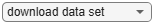true; if downloadDataset helperDownloadFiles('dataSet'); else helperGenerateCSINetDataset();%#ok end```
```Starting download data set. Downloading data set. Extracting files. Extract complete. ```
`load(fullfile(pwd,"processedData","info.mat"));`

Use the helperComputeLinkErrorRateWithPrecoder function to simulate a MIMO CDL channel in the frequency domain with a zero-forcing precoder. It uses the `importedCSINet` object to compress and decompress the estimated OFDM channel response and creates a precoder matrix based on CSINet channel estimates. It then computes the error rate between the transmitted and received information bits. Use 16-QAM modulation to view the effect of channel feedback compression with CSINet on the received constellation after applying ZF precoding. The random number generator seed is fixed for reproducibility purposes only.

```rng(112,'twister') bitsPerSymbol =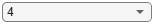4; opt.bps = bitsPerSymbol; opt.NumStreams = opt.NumRxAntennas; [errorRate, rxSymbols] = helperComputeLinkErrorRateWithPrecoder(importedCSINet,channel,carrier,opt); fprintf("Error rate on the CDL channel is %f \n",errorRate)```
```Error rate on the CDL channel is 0.432958 ```

Use a `comm.ConstellationDiagram` object to visualize the received symbols constellation. The constellation diagram shows that the received symbols can not be resolved with low error rate.

```refConstellation = qammod((0:2^bitsPerSymbol-1)',2^bitsPerSymbol,"UnitAveragePower",true); scope = comm.ConstellationDiagram( ... ShowReferenceConstellation=true, ... ReferenceConstellation=refConstellation, ... Title="Received Symbols", ... Name="Zero-Forcing Precoding with CSINet"); scope(rxSymbols(:))```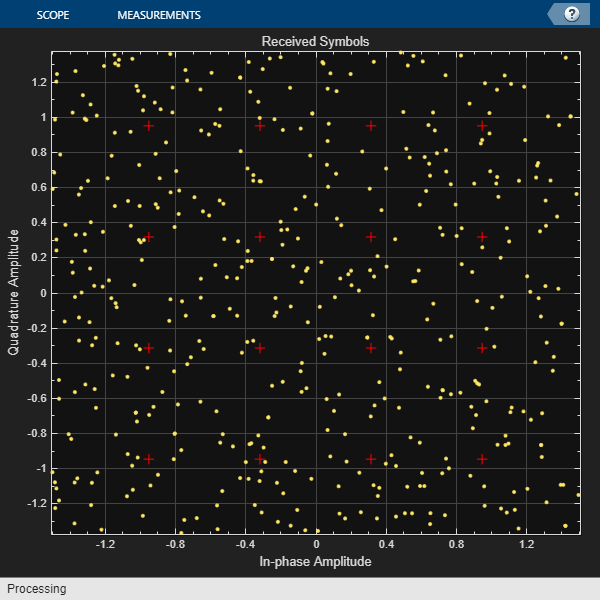Next, you fine-tune the weights of the imported autoencoder to the CDL channel model and evaluate the link performance.

### Fine-Tune Imported Model

The downloaded data set includes preprocessed training and validation data sets generated for a CDL channel with the same parameters listed in the previous section. The training and validation data sets are saved as MAT-files with the prefixes `CDLChannelEst_train` and `CDLChannelEst_val,` respectively. Read the data using a `signalDatastore` object and load it into memory using `readall`.

```trainSds = signalDatastore(fullfile(pwd,"processedData","CDLChannelEst_train*")); HTrainRealCell = readall(trainSds); HTrainReal = cat(1,HTrainRealCell{:}); HTrainReal = permute(HTrainReal,[2,3,4,1]); valSds = signalDatastore(fullfile(pwd,"processedData","CDLChannelEst_val*")); HValRealCell = readall(valSds); HValReal = cat(1,HValRealCell{:}); HValReal = permute(HValReal,[2,3,4,1]);```

Transfer learning is a technique that allows you to use a pretrained network on a new dataset without the need of training it from scratch. The weights of the `importedCSINet` are used as an initial point from which the Adam algorithm aims to find a better set of weights to compress and decompress the CDL channel estimates.

Use the `trainingOptions` function to set the hyper parameters for transfer learning. Use the `trainnet` function with the created `options` object and the `importedCSINet` object to train the CSINet model for 8 epochs (The imported CSINet was trained for one thousand epochs). You can use` trainnet` with built-in loss functions or a custom loss function (see `trainnet` (Deep Learning Toolbox)). To help the training converge faster, use the `normalizedMSEdB` helper as the loss function.

Stop the training early after 8 epochs to run the example quickly. To reduce the achieved error rate, set the `stopTrainingEarly` flag to `false` and allow the network to train for 100 epochs. Training the imported network for 100 epochs on NVIDIA GPU GeForce RTX 3080 takes about 5 minutes.

```gpurng(12) stopTrainingEarly =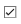true; if stopTrainingEarly epochs = 8; else epochs = 100;%#ok end options = trainingOptions("adam", ... InitialLearnRate=4e-3, ... MiniBatchSize=400, ... MaxEpochs=epochs, ... OutputNetwork="best-validation-loss", ... Shuffle="every-epoch", ... ValidationData={HValReal,HValReal}, ... LearnRateSchedule="piecewise", ... LearnRateDropPeriod=15, ... LearnRateDropFactor=0.8, ... ValidationPatience=10, ... ExecutionEnvironment="gpu", ... Plots="none", ... Verbose=1); net = trainnet(HTrainReal,HTrainReal,importedCSINet,@(x,t)normalizedMSEdB(x,t),options);```
``` Iteration Epoch TimeElapsed LearnRate TrainingLoss ValidationLoss _________ _____ ___________ _________ ____________ ______________ 0 0 00:00:03 0.004 -24.318 1 1 00:00:03 0.004 -23.398 50 2 00:00:14 0.004 -26.361 -26.287 100 4 00:00:24 0.004 -29.235 -28.33 150 6 00:00:34 0.004 -31.049 -31.032 200 8 00:00:43 0.004 -32.376 -32.035 208 8 00:00:46 0.004 -31.618 -31.231 Training stopped: Max epochs completed ```

Evaluate the performance of the model after transfer learning. The error rate drops to $<0.2$ after training the network on the new channel for only 8 epochs.

```[errorRate, rxSymbols] = helperComputeLinkErrorRateWithPrecoder(net,channel,carrier,opt);%#ok fprintf("Error rate after transfer learning is %f \n",errorRate)```
```Error rate after transfer learning is 0.162776 ```

Training for 100 epochs achieves error rate of approximately 0.05 with the received constellation shown below. The diagram shows that the received symbols resembles a noisy 16-QAM constellation after fine-tuning the model to compress the CDL channel feedback.

`% scope(rxSymbols(:))`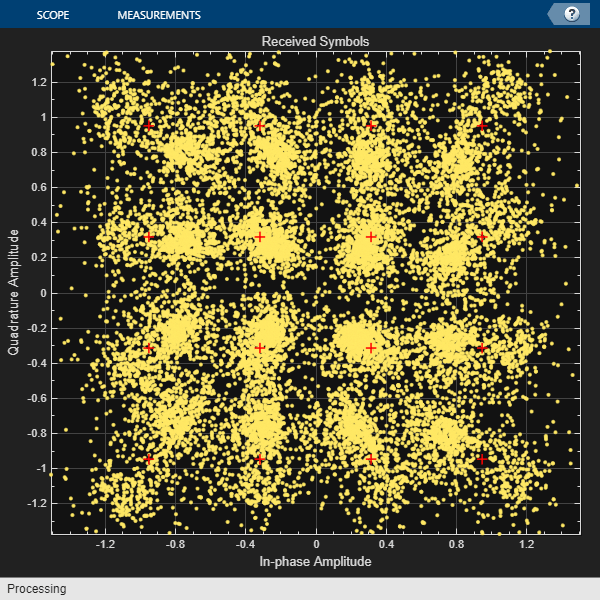### Analyze Trained Model for Codegen

Create a `coder.gpuEnvConfig` (GPU Coder) object and set the `DeepCodegen` property to `true`. Use the `coder.checkGpuInstall` (GPU Coder) function to verify that the compilers and libraries necessary for generating code using the NVIDIA cuDNN library are set up correctly. Set `DeepLibTarget` to `tensorrt` to use the tensorRT library instead.

```envCfg = coder.gpuEnvConfig("host"); envCfg.DeepLibTarget = "cudnn"; envCfg.DeepCodegen = 1; envCfg.Quiet = 0; coder.checkGpuInstall(envCfg);```
```Compatible GPU : PASSED CUDA Environment : PASSED Runtime : PASSED cuFFT : PASSED cuSOLVER : PASSED cuBLAS : PASSED cuDNN Environment : PASSED Host Compiler : PASSED Deep Learning (cuDNN) Code Generation: PASSED ```

Use `analyzeNetworkForCodegen` (GPU Coder) to check if the trained model has any incompatibilities for code generation.

`codegenSupport = analyzeNetworkForCodegen(net);`
``` Supported LayerDiagnostics _________ _________________________________________________________ none "No" "Found 1 issue(s) in 2 layer(s). View layer diagnostics." arm-compute "No" "Found 1 issue(s) in 2 layer(s). View layer diagnostics." mkldnn "No" "Found 1 issue(s) in 2 layer(s). View layer diagnostics." cudnn "No" "Found 1 issue(s) in 2 layer(s). View layer diagnostics." tensorrt "No" "Found 1 issue(s) in 2 layer(s). View layer diagnostics." ```

`analyzeNetworkForCodegen` (GPU Coder) found an issue with two layers in the autoencoder model. To fix the issue, use the `codegenSupport` struct to `v`iew the diagnostics message.

`codegenSupport(4).LayerDiagnostics`
```ans=2×3 table LayerName LayerType Diagnostics ___________ ______________________________________ _______________________________________________________________________________________________________________________________________________________ "reshape_1" "CSINetOutdoor64.kReshape1Layer300021" "Unsupported custom layer 'kReshape1Layer300021'. Code generation does not support custom layers without '%#codegen' defined in the class definition. " "reshape" "CSINetOutdoor64.kReshapeLayer299964" "Unsupported custom layer 'kReshapeLayer299964'. Code generation does not support custom layers without '%#codegen' defined in the class definition. " ```

Layers `reshape` and `reshape_1` are automatically generated custom layers translated from the imported TensorFlow model. The translated layers do not support code generation based on the diagnostics for target library `cuDNN`. Use `helperRowMajorReshapeLayer` to replace the imported reshape layers with a custom row-major reshape layer that matches the default reshape operation in TensorFlow.

```rowMajorReshape = helperRowMajorReshapeLayer(2*32*32,1,1,"row-major reshape"); lgraph = replaceLayer(layerGraph(net),"reshape",rowMajorReshape); rowMajorReshape1 = helperRowMajorReshapeLayer(2,32,32,"row-major reshape_1"); lgraph = replaceLayer(lgraph,"reshape_1", rowMajorReshape1); CSINet = dlnetwork(lgraph); codegenSupport = analyzeNetworkForCodegen(CSINet);```
``` Supported _________ none "Yes" arm-compute "Yes" mkldnn "Yes" cudnn "Yes" tensorrt "Yes" ```

Save the `dlnetwork` object, `CSINet`, to a MAT-File for code generation next.

`save CDL_CSINet.mat CSINet`

Create an entry-point function, `CSINet_predict`, that takes the channel estimate matrix, `Hest`, runs prediction on `CSINet`, and returns `Hest_hat`. `CSINet_predict` loads the trained model as a persistent variable once using `coder.loadDeepLearningNetwork` (GPU Coder) and uses it in subsequent calls.

`type("CSINet_predict.m")`
```function Hest_hat = CSINet_predict(Hest) persistent mynet; if isempty(mynet) mynet = coder.loadDeepLearningNetwork('CDL_CSINet.mat', 'CSINet'); end Hest_hat = predict(mynet,dlarray(Hest,'SSC')); ```

### Generate C++ Code

Use the `coder.gpuConfig` (GPU Coder) object with `build_type` set to `"mex"` to test the generated code from MATLAB. Set the `TargetLibrary` to `cudnn` and use the `codegen` (MATLAB Coder) command to generate C++ code from the entry point function `CSINet_predict`.

```cfg = coder.gpuConfig("mex"); cfg.TargetLang = "C++"; cfg.DeepLearningConfig = coder.DeepLearningConfig("TargetLibrary","cudnn"); codegen -config cfg CSINet_predict -o CSINet_predict_cudnn -args {coder.typeof(single(0),[32 32 2])} -report```
```Code generation successful: View report ```

To test the accuracy of the generated C++ code, compute the error rate using the generated MEX function, `CSINet_predict_cudnn`.

```[errorRateMEX, rxSymbols] = helperComputeLinkErrorRateWithPrecoder(@(x) CSINet_predict_cudnn(x),channel,carrier,opt); fprintf("Error rate using CSINet CUDA code is %f \n",errorRateMEX)```
```Error rate using CSINet CUDA code is 0.162807 ```
`fprintf("Variance in error rate after codegen is %f \n",abs(errorRate-errorRateMEX))`
```Variance in error rate after codegen is 0.000031 ```

Clear the static network object that was loaded in memory.

`clear mex;`

### Further Exploration

In this example, you imported a pretrained TensorFlow model, tested the model in a link simulation, and fine-tuned its weights to a new channel model. You generated C++ code to target the NVIDIA GPU using cuDNN library. Finally, you compared the achieved error rate by using generated CUDA code for autoencoder channel feedback model to that computed in native MATLAB simulation. The variance in error rate for the pretrained model used in this example is < 1e-4.

Try using a pretrained model with a lower compression rate from the repository in  and test its performance before and after transfer learning. A lower compression rate, for example, 1/4, results in a lower error rate after training for the same number of epochs. Tune the training hyper parameters for each model to find the best set of weights for the autoencoder model. Vary the QAM modulation order or replace the ZF precoder with an MMSE precoder and test the performance of the autoencoder model. Set the `coder.DeepLearningConfig` (GPU Coder) object to use NVIDIA TensorRT deep learning library instead of cuDNN and test the accuracy of the generated C++ code. You can configure the code generator to take advantage of the TensorRT library precision modes (fp32, fp16, or int8) for inference. Low precision accelerates inference and optimizes memory requirements at the expense of slightly lower accuracy. For details, see the `coder.TensorRTConfig` (GPU Coder) object.

### Local Functions

```function nmse = normalizedMSEdB(HTest, HhatCSINet) % Calculate normalized MSE between test & predicted channel estimates in dB HTestComplex= complex(HTest(:,:,1,:),HTest(:,:,2,:)); power = sum(abs(HTestComplex).^2, [1 2]); nmse = 10.*log(mean(sum(abs(HTest - HhatCSINet).^2, [1,2,3])./power))./log(10); end function helperDownloadFiles(target) % Download CDL Channel model data set or pretrained TensorFlow model if strcmp(target,'dataSet') targetDir = 'coexecutionPrecoding/processedData'; name = 'data set'; elseif strcmp(target,'pretrainedModel') targetDir = 'importCSINetTensorFlow/tensorflowModel/CSINetOutdoor64'; name = 'pretrained model'; end dstFolder = pwd; downloadAndExtractDataFile(targetDir,dstFolder,name,target); end function downloadAndExtractDataFile(targetDir,dstFolder,name,target) if strcmp(target,'dataSet') folderName = 'processedData'; elseif strcmp(target,'pretrainedModel') folderName = 'CSINetOutdoor64'; end if exist(folderName,"dir") fprintf([folderName,' folder exists. Skip download.\n\n']); else fprintf(['Starting download ',name,'.\n']) fileFullPath = matlab.internal.examples.downloadSupportFile('spc/', ... [targetDir,'.zip']); fprintf(['Downloading ',name,'. Extracting files.\n']) unzip(fileFullPath,dstFolder); fprintf('Extract complete.\n\n') end end```

### References

 Chao-Kai Wen, Wan-Ting Shih, and Shi Jin, "Deep learning for massive MIMO CSI feedback," IEEE Wireless Communications Letters, Vol. 7, No. 5, pp. 748–751, Oct. 2018.

 3GPP TR 38.901. “Study on channel model for frequencies from 0.5 to 100 GHz.” 3rd Generation Partnership Project; Technical Specification Group Radio Access Network.